# Cost Function and Optimal β

In this blog, we will discuss the cost function for linear regression.

The cost function is simply a major of error or loss of a model based on the input dataset and takes both actual and predicted data into consideration while calculating error. The ultimate goal of any machine learning model is to find optimal coefficients which can minimize the error of cost function.

In the case of Linear regression, RSS is the cost function and we have to minimize the cost function to find the optimal value of β0 and β1.

#### The cost function for linear regression:The cost function can be minimized using:

• Differentiation

In this module, we will use the differentiation method and calculate to derive β0 and β1.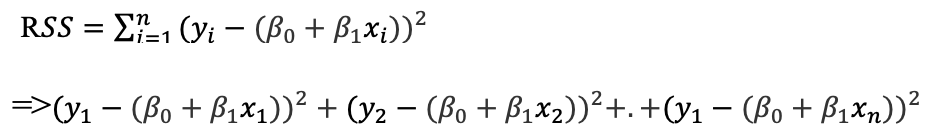Expand the equation: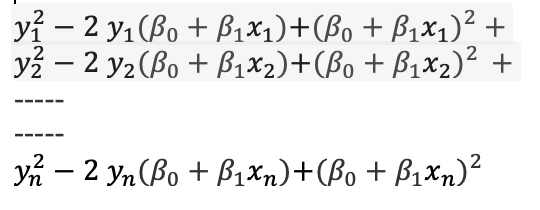Expand remaining terms.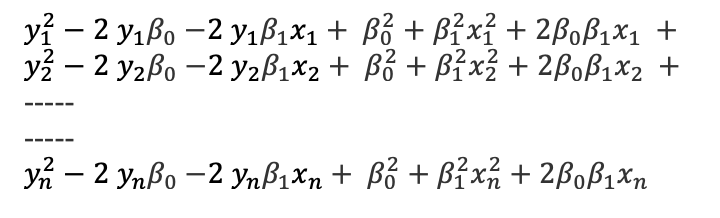Group all similar components.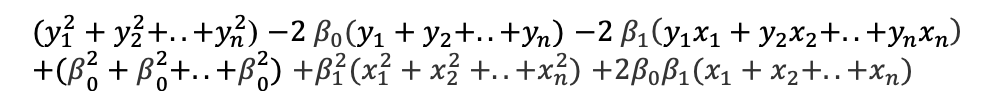Now let’s understand each component. We can re-write as below.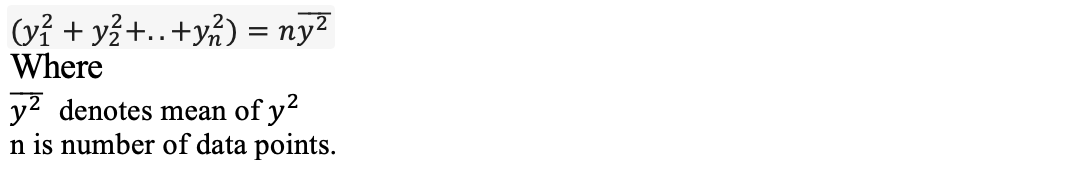Similarly, we can re-write each component as below.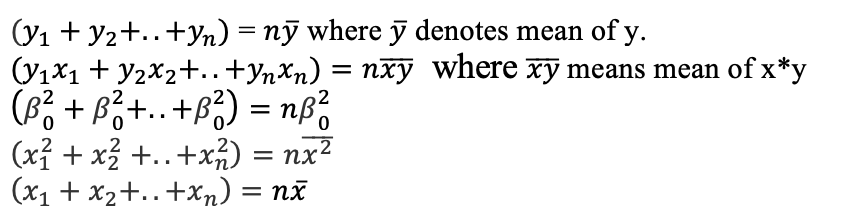Replace the above notations and rewrite our equation: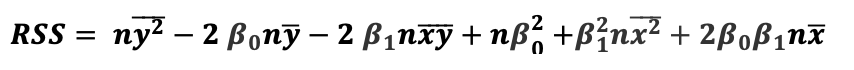To minimize and find the optimal value for β0 and β1, we need to perform partial derivation with respect to β0 and β1.

We need to compute below partial derivatives and equate it to zero.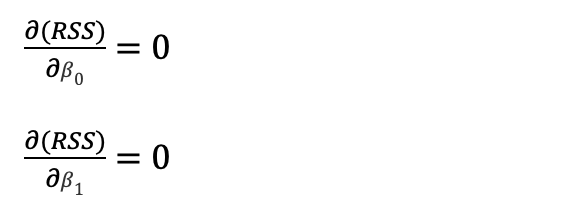Let’s calculate the partial derivation of both equations: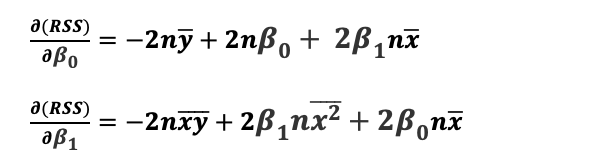At this point, we have two equations and two variables. Solving both equations we can find the value of β0 and β1.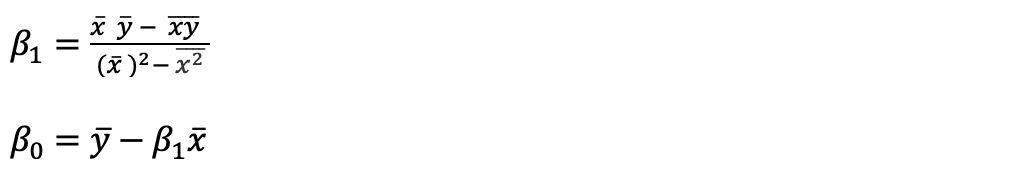Now that we have found β0 and β1. Let’s use our earlier example and find out the optimal values for the best-fit line.

Here are the data points for which we are trying to find the best-fit line.

 ad_spending Sales (In thousands) 1000 139 1500 155 1800 160 2000 210 800 120 1200 150 1400 145 1700 164 1100 125

Upon calculating, we find the below details.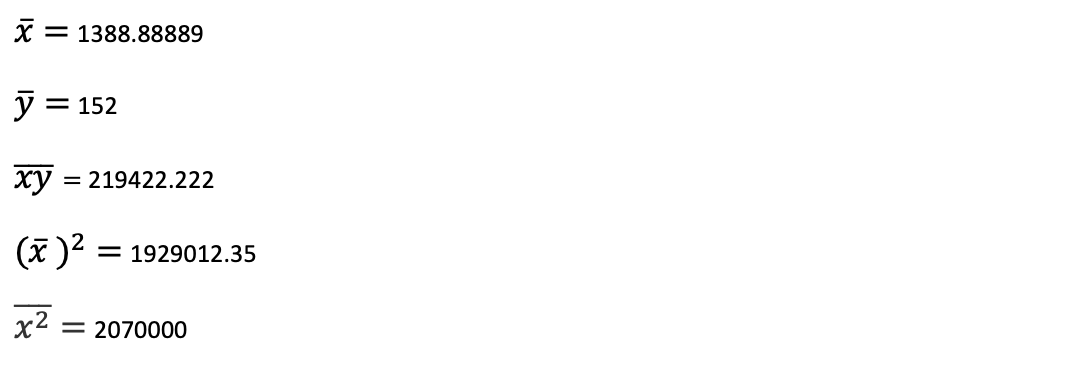Replace these values to find β1.

β≈ 0.06

β0 ≈ 69

So the final equation of the best fit line is

y=69+0.06x

Plot the best-fit line to verify the graph.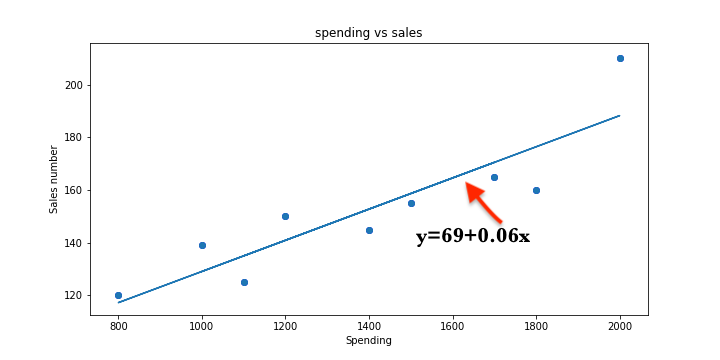Conclusion:

You have understood about Linear Regression cost function and how to find Optimal β.  Follow this link to find more about Linear Regression.